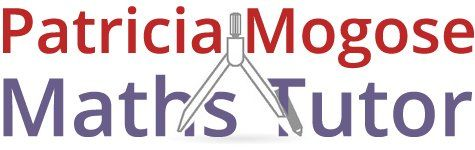For maths tuition with Patricia, call:
01733 265 440
patricia.mogose@sky.com

#### BSc Honours and PGCE qualified# Maths tutoring services for primary school studentsin Peterborough

Bring out your child’s true potential with reliable maths tutoring services. At Patricia Mogose Maths Tutor in Peterborough, I offer professional tuition for primary school, secondary school and A-level students. Contact me today.### Year 3 students

• Multiples of numbers
• More fractions: 1/8, 1/10 etc.
• Decimal fractions: getting a feel for their size
• Continued use of the 4 main operators: +, -, x, / and extending to more detailed calculations
• introducing symbols: less than <, and greater than >
• Adding more than 2 numbers
• Multiplying 2 digits by 1 digit
• Introducing the larger times tables to 10
• Division: halving and quartering by division
• Understanding concept of remainder in division
• Square numbers and prime numbers### Tuition for year 4 students

• Odds and Evens: Telling whether the result of an addition is odd or even by looking at the properties of the two numbers added together
• Introducing ratios
• Decimals and Fractions, looking at their relationship
• Subtracting decimals
• Multiplication by doubling
• Division by a single digit
• Extending work with the 4 operators
• Measurement in different units
• Negative numbers appreciation of ‘more’ and ‘less’
• Areas by counting squares
• Perimeters of shapes
• 3D shapes: faces, edges, vertices, nets
• Angles and measurement by protractor
• Compass points
• Fraction equivalence### Year 5 maths tuition

• Writing larger numbers as figures and words
• Estimating calculations by rounding
• Estimating fractions of quantities
• Negative numbers: finding the difference between 2 such numbers
• Multiples and Factors
• Number squaring
• Fractions: comparison of, with different denominators
• Considering one quantity as a fraction of another
• Percentage of a quantity, calculation on paper
• Further extension of work with the 4 operators
• Adding various sized numbers in appropriate columns
• Subtracting: hundreds and thousands
• Multiplying of decimals and long multiplication
• Dividing: into numbers in hundreds and thousands
• Calculator work for all operations, including more complex problem solving
• Areas in square mm, cm, m, also converting between such units
• Metric and imperial units: appreciation and comparison
• Triangles: identifying the different types
• Time: the 24-hour clock
• Types of angles and calculating a missing angle
• Transformations: Reflection and Rotation
• Probability: appreciation of, and associated words### Year 6 maths

• This year will be consolidation of work from previous years and new material. At the end of Year 6, SATs tests in arithmetical skills and mathematical reasoning will be taken.
• Describing the pattern in number sequences
• Awareness of larger multiples of single digit numbers
• Fractions: using all 4 operators, mixed numbers, improper fractions
• Percentages of quantities, one number as percentage of another, with and without calculator
• Polygons: names and properties
• Extending work with the 4 operators
• Long multiplication with 3 digit numbers
• Division: Long, by 2 digit numbers
• Estimating decimal calculations by rounding to a whole number
• Calculator work – extended to fractions
• Brackets in more complex calculations
• Converting metric/imperial units
• Area calculation: square, rectangle and triangle